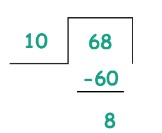# Divisibility Calculator

To use divisibility test calculator, enter the numbers and click the calculate button

Give Us Feedback

## Divisibility Calculator

Divisibility test calculator is used to check whether the number is divisible by another number or not. This calculator takes two numbers for testing the divisibility, it shows divisible if the number is divisible by another number otherwise it shows not divisible.

## What is the divisibility test?

The divisibility test is used to test whether the number is divisible by another number or not. For example, if you want to check a number like 2310 is divisible on 2, 3, 4, & 5, then you have to apply the conditions of the divisibility.

Dividing the number by another number is also used to check the divisibility. If the number is divided by the other number, then it is divisible by that number. If there is a remainder while dividing the number then that number is not divisible on another number.

## Conditions of divisibility

• A number is divisible by 2 if the last digit of the given number is 0, 2, 4, 6, or 8.
• A number is divisible by 3 if the sum of each digit of the number is divisible by 3.
• A number is divisible by 4 if the last two digits of the number are divisible by 4.
• A number is divisible by 5 if the last digit of the number is 0 or 5.
• A number is divisible by 6 if the number is divisible by 2 and 3.
• A number is divisible by 9 if the sum of each digit of the number is divisible by 9.
• A number is divisible by 10 if the last digit of the number is 0.

Many other conditions are also used for 12, 15, and 25 times tables. You can simply use the divisibility calculator to check the result according to the conditions of the divisibility test.

## How to check the divisibility of numbers?

Follow the below examples to learn how to check the divisibility of numbers.

Example 1

Is 78 is divisible by 2?

Solution

Check the divisibility of 78 by 2.

Divide the numbers.

78/2 = 39

As there is no remainder while dividing the number. So, 78 is divisible by 2.

Alternately

According to the condition of divisibility, a number must be divisible by 2 if the last digit of the number is 0 or an even number. As the last digit of the given number is even so the number is divisible by 2.

Example 2

Is 68 is divisible by 10?

Solution

Check the divisibility of 68 by 10.

Divide both the numberAs there is a remainder of 8 in this division. So, 68 is not divisible by 10.

Alternately

According to the condition of divisibility, a number must be divisible by 10 if the last digit of the number is 0. As you can see that the last digit of the number is not “0”. So, 68 is not divisible by 10.

### Math Tools